# NCERT Exemplar Class 10 Maths Solutions for Chapter 2 - Polynomials

## NCERT Exemplar Solutions Class 10 Maths Chapter 2 – Free PDF Download

NCERT Exemplar Class 10 Maths Chapter 2 Polynomials are provided here for students to prepare for the board exam. These solutions are prepared as per NCERT guidelines and the latest CBSE syllabus (2023-2024) by our subject experts. With the help of exemplar problems and solutions, students will be able to revise the complete chapter and score better marks in the exam.

In this chapter, students will learn to solve exemplar problems based on polynomials and their subtopics like:

• Geometrical Meaning of the Zeroes of a Polynomial
• Division Algorithm for Polynomial
• Zeroes and Coefficients of a Polynomial and Their Relationships

In NCERT Exemplar Class 10 Maths Chapter 2, students will learn about the concept of polynomials which has been discussed in detail. They will also study important topics like the relationship between coefficients and zeroes, the division algorithm for polynomials and the geometrical meaning of the zeroes of a polynomial. As students need to be thorough with these topics, free NCERT Exemplar for Chapter 2 – Polynomials is provided here.

Students can download the Class 10 Maths Chapter 2 NCERT Exemplar PDF from the link provided below.

## Download the PDF of NCERT Exemplar Solutions for Class 10 Maths Chapter 2 Polynomials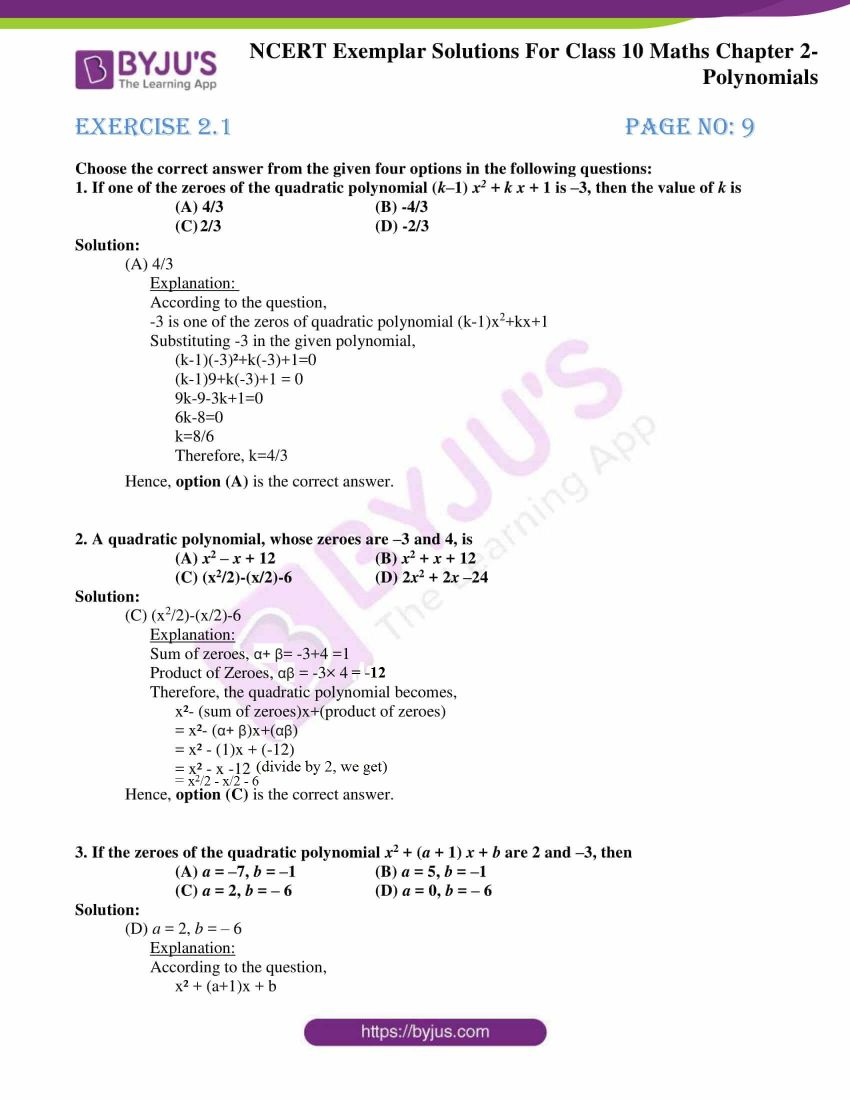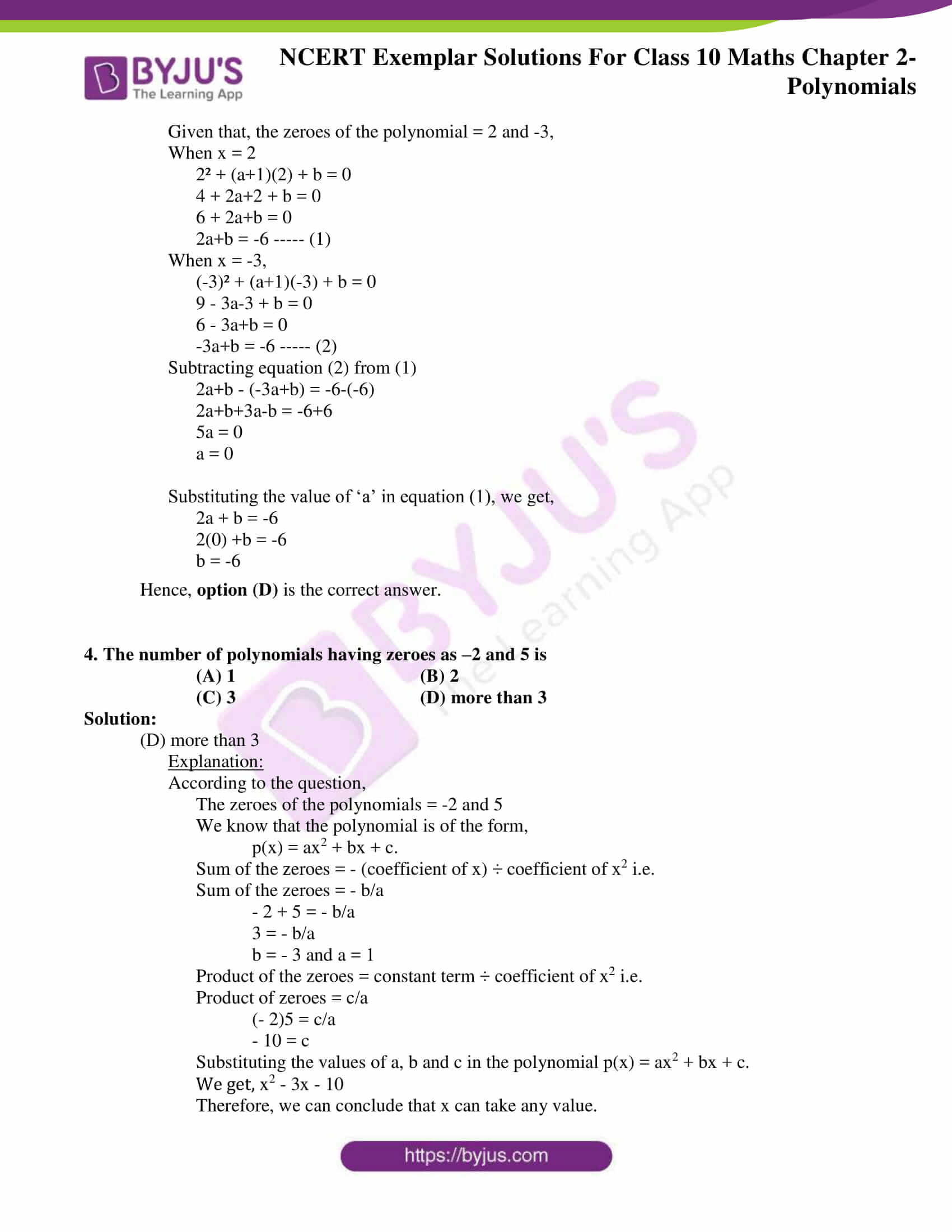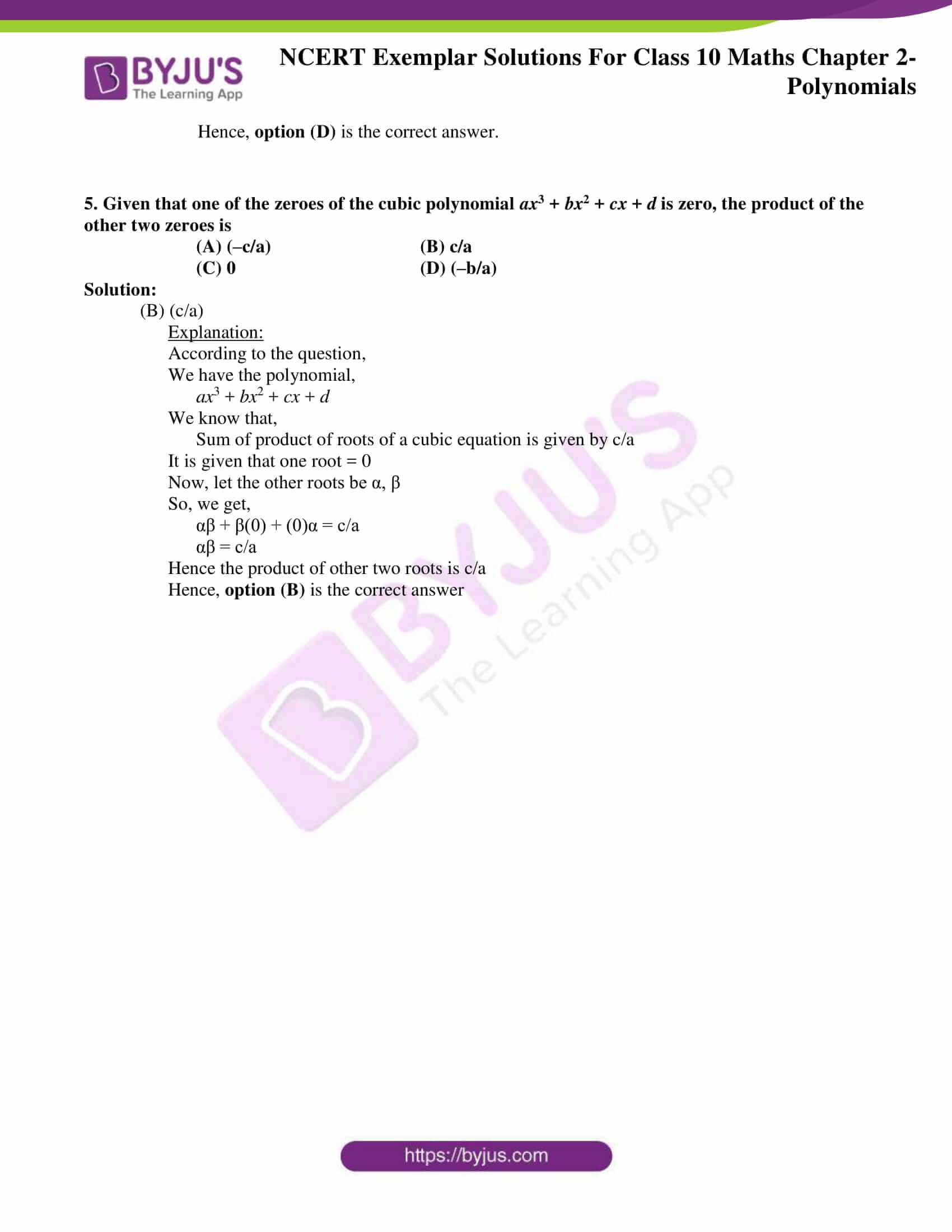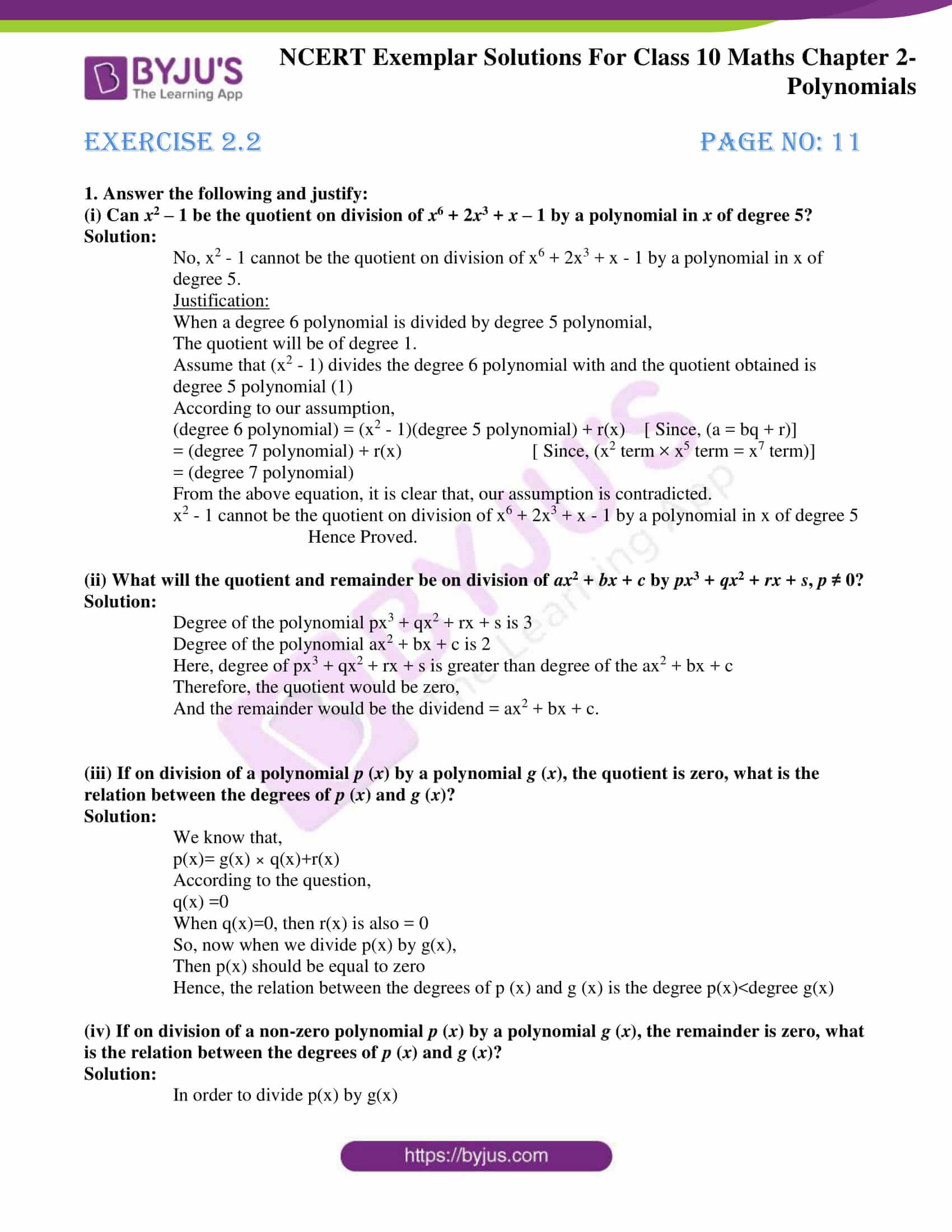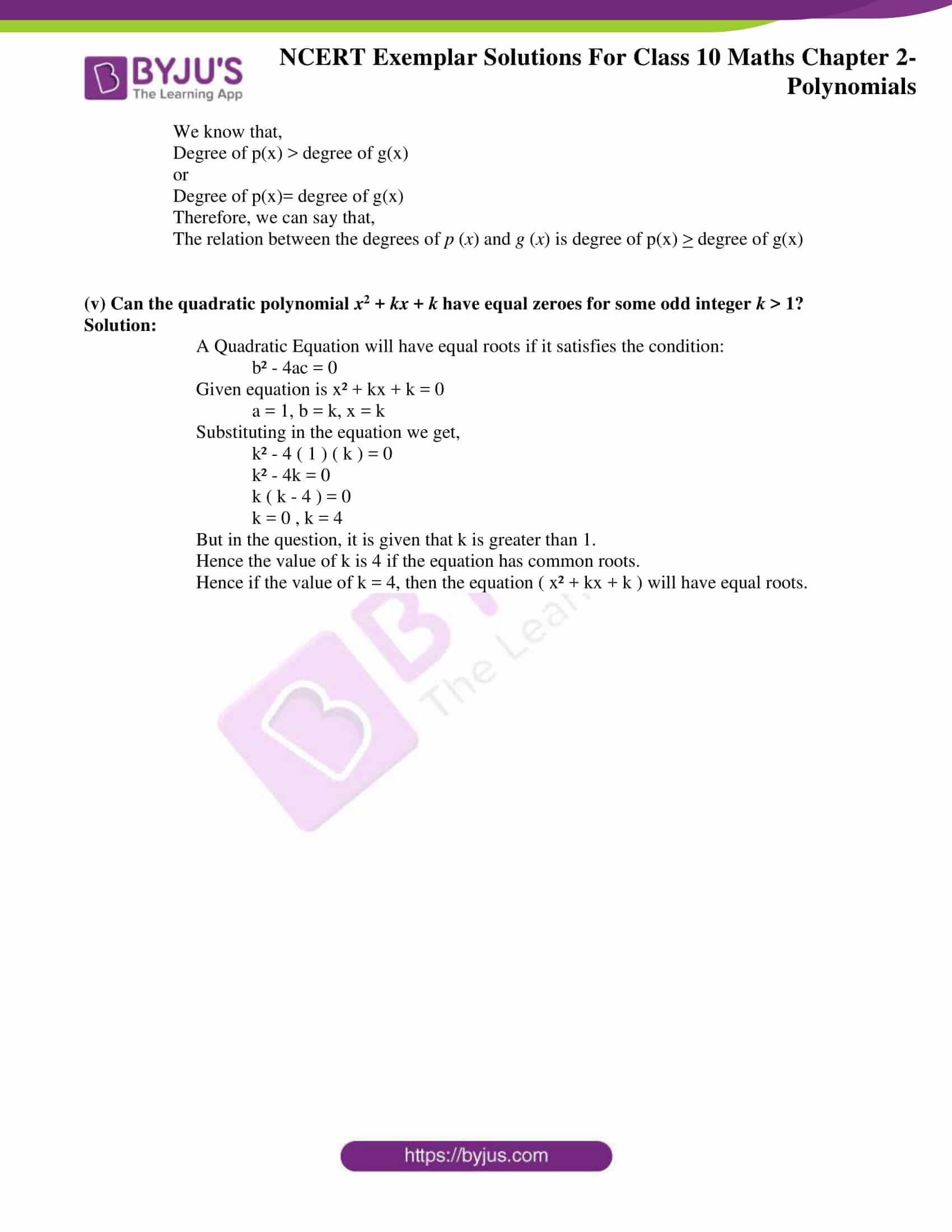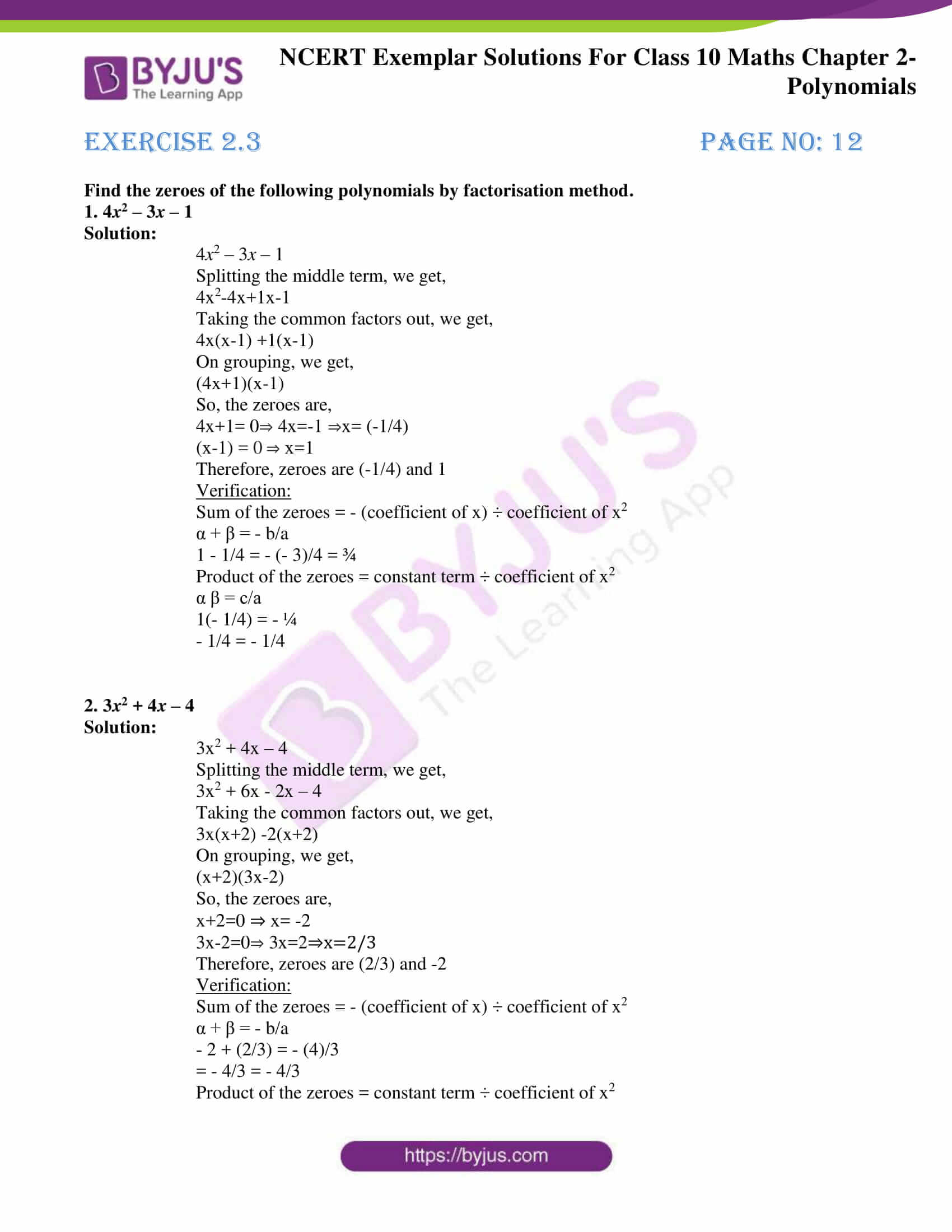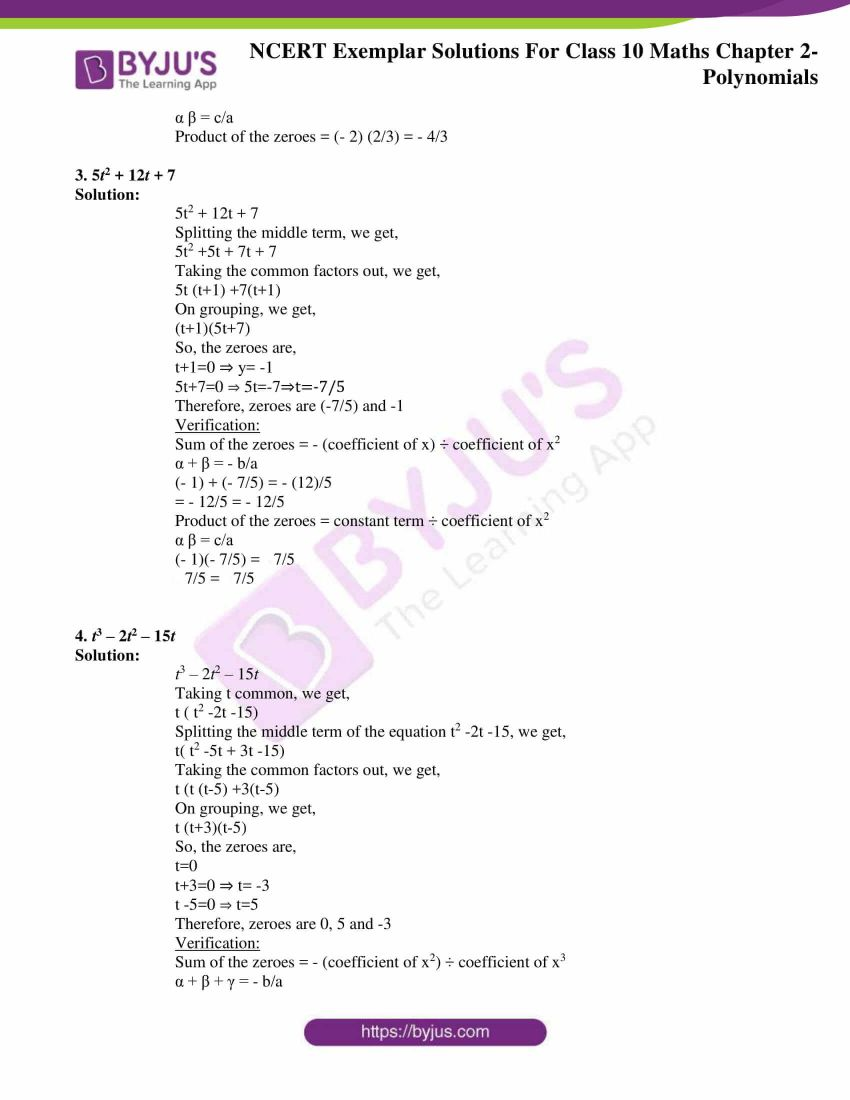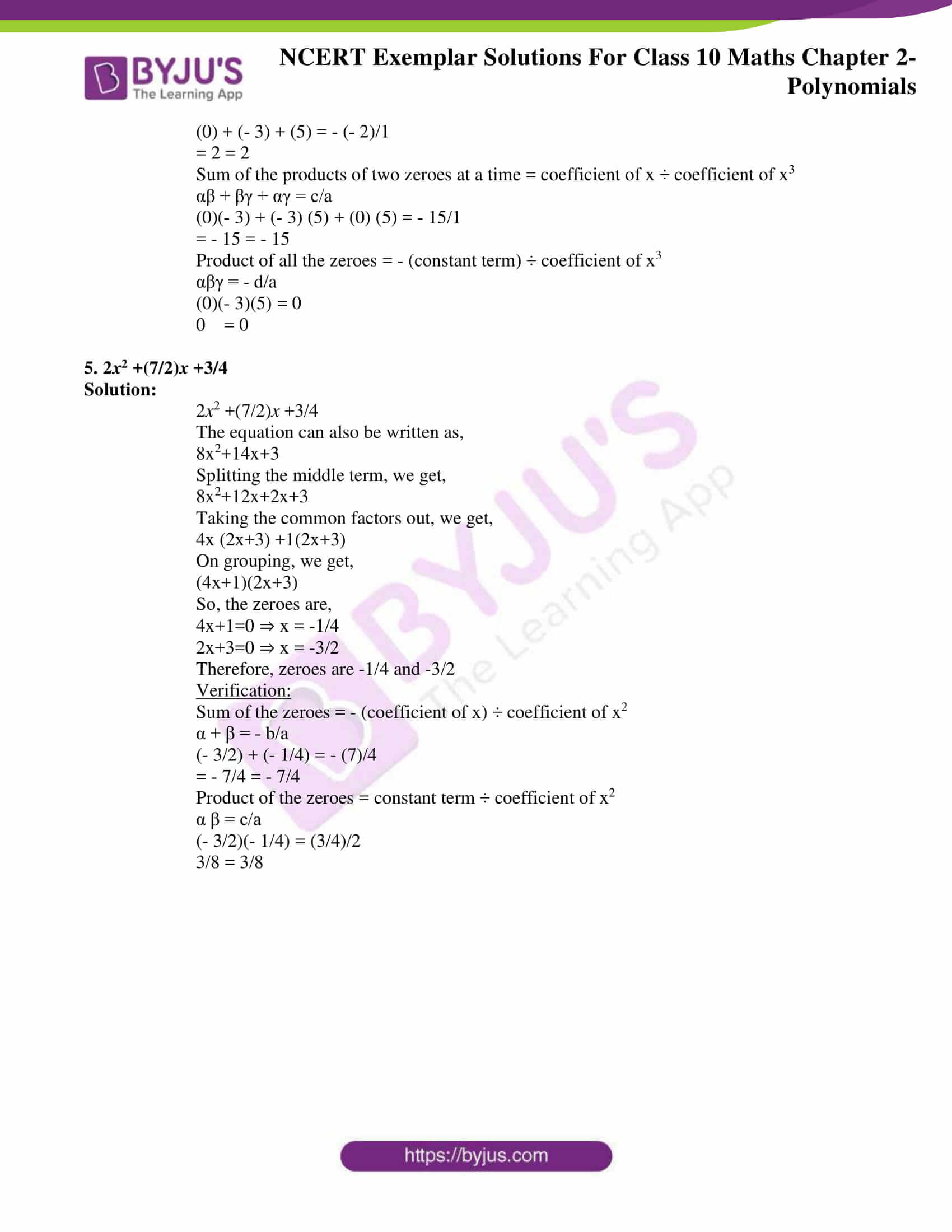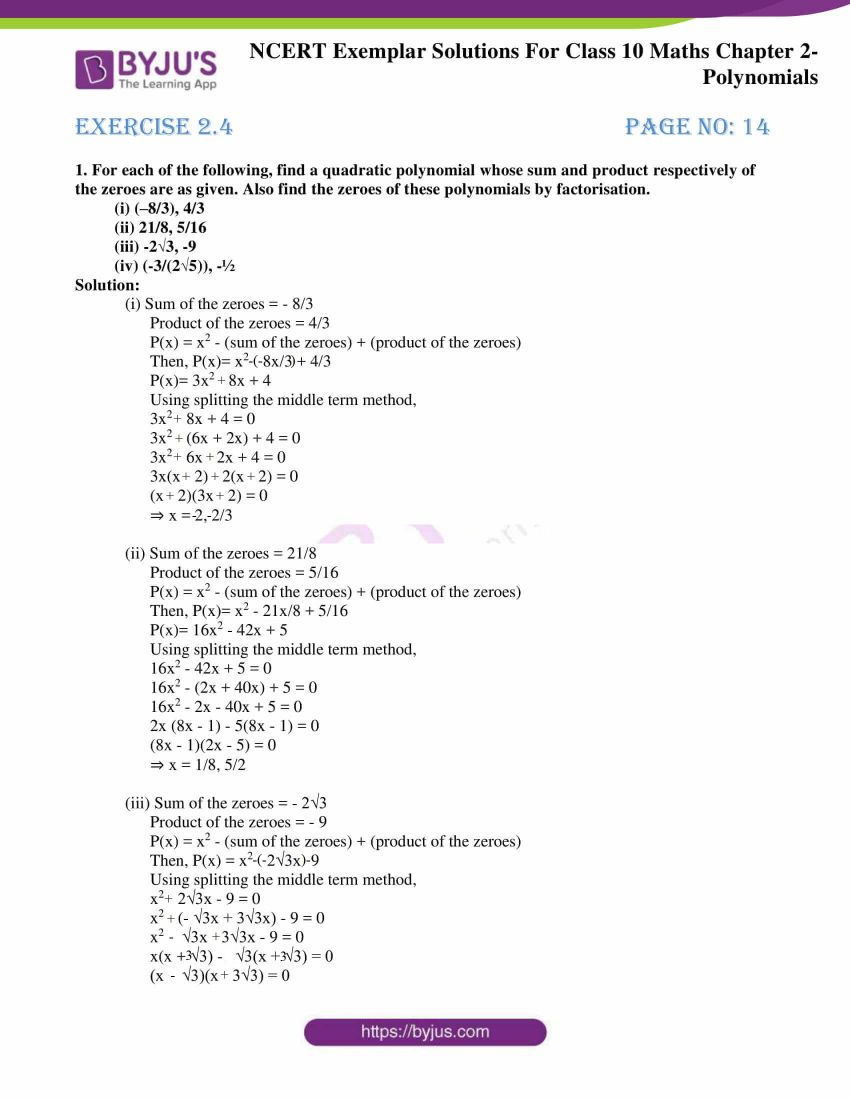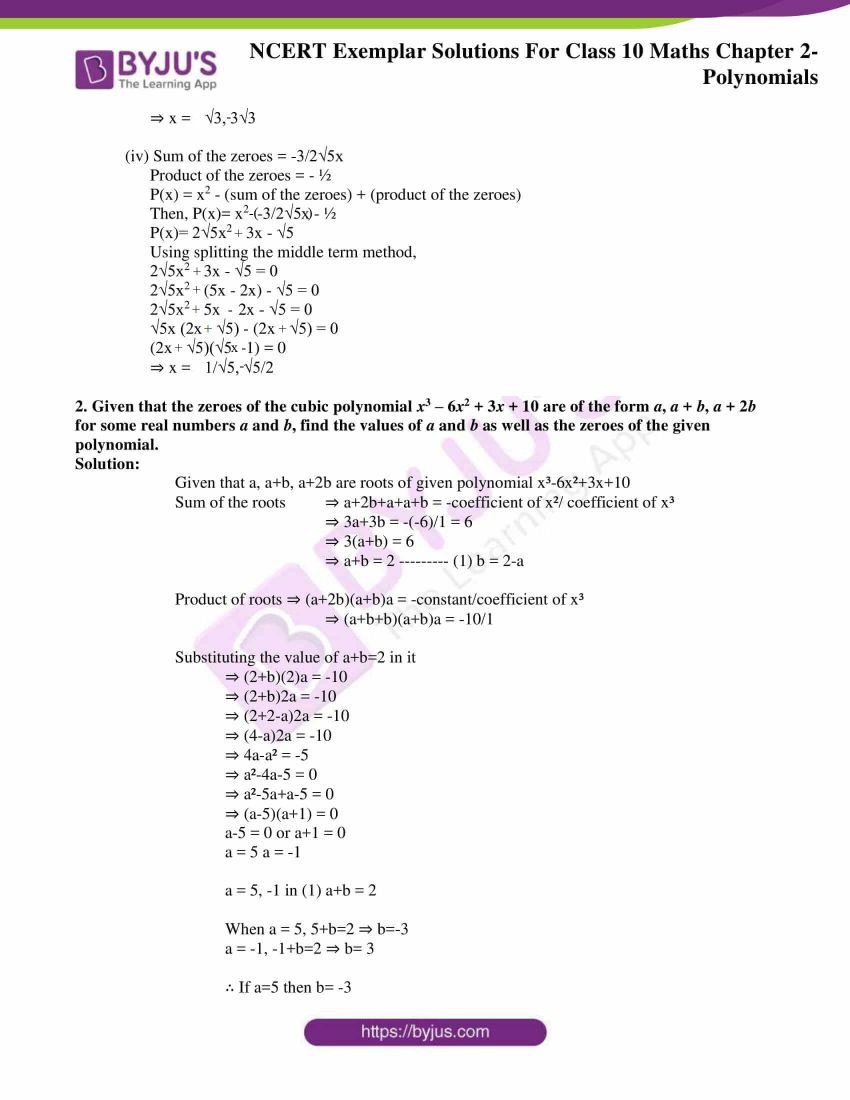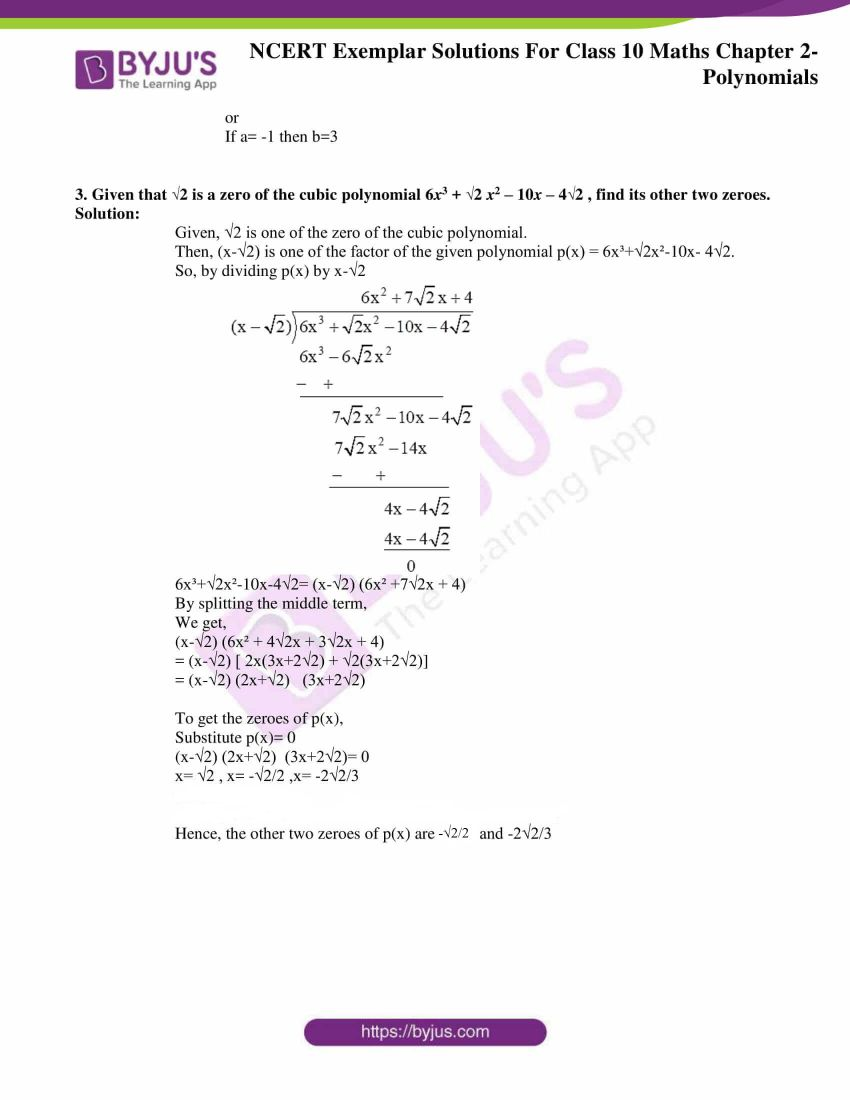## Exercise 2.1

Choose the correct answer from the given four options in the following questions:

1. If one of the zeroes of the quadratic polynomial (k–1) x2 + k x + 1 is –3, then the value of k is

(A) 4/3 (B) -4/3

1. 2/3 (D) -2/3

Solution:

(A) 4/3

Explanation:

According to the question,

-3 is one of the zeros of quadratic polynomial (k-1)x2+kx+1

Substituting -3 in the given polynomial,

(k-1)(-3)²+k(-3)+1=0

(k-1)9+k(-3)+1 = 0

9k-9-3k+1=0

6k-8=0

k=8/6

Therefore, k=4/3

Hence, option (A) is the correct answer.

2. A quadratic polynomial, whose zeroes are –3 and 4, is

(A) x2x + 12 (B) x2 + x + 12

(C) (x2/2)-(x/2)-6 (D) 2x2 + 2x –24

Solution:

(C) (x2/2)-(x/2)-6

Explanation:

Sum of zeroes, α+ β= -3+4 =1

Product of Zeroes, αβ = -3× 4 = -12

x²- (sum of zeroes)x+(product of zeroes)

= x²- (α+ β)x+(αβ)

= x² – (1)x + (-12)

= x² – x -12

divide by 2, we get

= x²/2 – x/2 -12/2

= x²/2 – x/2 -6

Hence, option (C) is the correct answer.

3. If the zeroes of the quadratic polynomial x2 + (a + 1) x + b are 2 and –3, then

(A) a = –7, b = –1 (B) a = 5, b = –1

(C) a = 2, b = – 6 (D) a = 0, b = – 6

Solution:

(D) a = 2, b = – 6

Explanation:

According to the question,

x² + (a+1)x + b

Given that, the zeroes of the polynomial = 2 and -3,

When x = 2

2² + (a+1)(2) + b = 0

4 + 2a+2 + b = 0

6 + 2a+b = 0

2a+b = -6 —– (1)

When x = -3,

(-3)² + (a+1)(-3) + b = 0

9 – 3a-3 + b = 0

6 – 3a+b = 0

-3a+b = -6 —– (2)

Subtracting equation (2) from (1)

2a+b – (-3a+b) = -6-(-6)

2a+b+3a-b = -6+6

5a = 0

a = 0

Substituting the value of ‘a’ in equation (1), we get,

2a + b = -6

2(0) +b = -6

b = -6

Hence, option (D) is the correct answer.

4. The number of polynomials having zeroes as –2 and 5 is

(A) 1 (B) 2

(C) 3 (D) more than 3

Solution:

(D) more than 3

Explanation:

According to the question,

The zeroes of the polynomials = -2 and 5

We know that the polynomial is of the form,

p(x) = ax2 + bx + c.

Sum of the zeroes = – (coefficient of x) ÷ coefficient of x2 i.e.

Sum of the zeroes = – b/a

– 2 + 5 = – b/a

3 = – b/a

b = – 3 and a = 1

Product of the zeroes = constant term ÷ coefficient of x2 i.e.

Product of zeroes = c/a

(- 2)5 = c/a

– 10 = c

Substituting the values of a, b and c in the polynomial p(x) = ax2 + bx + c.

We get, x2 – 3x – 10

Therefore, we can conclude that x can take any value.

Hence, option (D) is the correct answer.

5. Given that one of the zeroes of the cubic polynomial ax3 + bx2 + cx + d is zero, the product of the other two zeroes is

(A) (–c/a) (B) c/a

(C) 0 (D) (–b/a)

Solution:

(B) (c/a)

Explanation:

According to the question,

We have the polynomial,

ax3 + bx2 + cx + d

We know that,

Sum of product of roots of a cubic equation is given by c/a

It is given that one root = 0

Now, let the other roots be α, β

So, we get,

αβ + β(0) + (0)α = c/a

αβ = c/a

Hence the product of other two roots is c/a

Hence, option (B) is the correct answer

## Exercise 2.2

1. Answer the following and justify:

(i) Can x2 – 1 be the quotient on division of x6 + 2x3 + x – 1 by a polynomial in x of degree 5?

Solution:

No, x2 – 1 cannot be the quotient on division of x6 + 2x3 + x – 1 by a polynomial in x of degree 5.

Justification:

When a degree 6 polynomial is divided by degree 5 polynomial,

The quotient will be of degree 1.

Assume that (x2 – 1) divides the degree 6 polynomial with and the quotient obtained is degree 5 polynomial (1)

According to our assumption,

(degree 6 polynomial) = (x2 – 1)(degree 5 polynomial) + r(x) [ Since, (a = bq + r)]

= (degree 7 polynomial) + r(x) [ Since, (x2 term × x5 term = x7 term)]

= (degree 7 polynomial)

From the above equation, it is clear that, our assumption is contradicted.

x2 – 1 cannot be the quotient on division of x6 + 2x3 + x – 1 by a polynomial in x of degree 5

Hence Proved.

(ii) What will the quotient and remainder be on division of ax2 + bx + c by px3 + qx2 + rx + s, p ≠ 0?

Solution:

Degree of the polynomial px3 + qx2 + rx + s is 3

Degree of the polynomial ax2 + bx + c is 2

Here, degree of px3 + qx2 + rx + s is greater than degree of the ax2 + bx + c

Therefore, the quotient would be zero,

And the remainder would be the dividend = ax2 + bx + c.

(iii) If on division of a polynomial p (x) by a polynomial g (x), the quotient is zero, what is the relation between the degrees of p (x) and g (x)?

Solution:

We know that,

p(x)= g(x) × q(x)+r(x)

According to the question,

q(x) =0

When q(x)=0, then r(x) is also = 0

So, now when we divide p(x) by g(x),

Then p(x) should be equal to zero

Hence, the relation between the degrees of p (x) and g (x) is the degree p(x)<degree g(x)

(iv) If on division of a non-zero polynomial p (x) by a polynomial g (x), the remainder is zero, what is the relation between the degrees of p (x) and g (x)?

Solution:

In order to divide p(x) by g(x)

We know that,

Degree of p(x) > degree of g(x)

or

Degree of p(x)= degree of g(x)

Therefore, we can say that,

The relation between the degrees of p (x) and g (x) is degree of p(x) > degree of g(x)

(v) Can the quadratic polynomial x2 + kx + k have equal zeroes for some odd integer k > 1?

Solution:

A Quadratic Equation will have equal roots if it satisfies the condition:

b² – 4ac = 0

Given equation is x² + kx + k = 0

a = 1, b = k, x = k

Substituting in the equation we get,

k² – 4 ( 1 ) ( k ) = 0

k² – 4k = 0

k ( k – 4 ) = 0

k = 0 , k = 4

But in the question, it is given that k is greater than 1.

Hence the value of k is 4 if the equation has common roots.

Hence if the value of k = 4, then the equation ( x² + kx + k ) will have equal roots.

## Exercise 2.3

Find the zeroes of the following polynomials by factorisation method.

1. 4x2 – 3x – 1

Solution:

4x2 – 3x – 1

Splitting the middle term, we get,

4x2-4x+1x-1

Taking the common factors out, we get,

4x(x-1) +1(x-1)

On grouping, we get,

(4x+1)(x-1)

So, the zeroes are,

4x+1= 0⇒ 4x=-1 ⇒x= (-1/4)

(x-1) = 0 ⇒ x=1

Therefore, zeroes are (-1/4) and 1

Verification:

Sum of the zeroes = – (coefficient of x) ÷ coefficient of x2

α + β = – b/a

1 – 1/4 = – (- 3)/4 = ¾

Product of the zeroes = constant term ÷ coefficient of x2

α β = c/a

1(- 1/4) = – ¼

– 1/4 = – 1/4

2. 3x2 + 4x – 4

Solution:

3x2 + 4x – 4

Splitting the middle term, we get,

3x2 + 6x – 2x – 4

Taking the common factors out, we get,

3x(x+2) -2(x+2)

On grouping, we get,

(x+2)(3x-2)

So, the zeroes are,

x+2=0 ⇒ x= -2

3x-2=0⇒ 3x=2⇒x=2/3

Therefore, zeroes are (2/3) and -2

Verification:

Sum of the zeroes = – (coefficient of x) ÷ coefficient of x2

α + β = – b/a

– 2 + (2/3) = – (4)/3

= – 4/3 = – 4/3

Product of the zeroes = constant term ÷ coefficient of x2

α β = c/a

Product of the zeroes = (- 2) (2/3) = – 4/3

3. 5t2 + 12t + 7

Solution:

5t2 + 12t + 7

Splitting the middle term, we get,

5t2 +5t + 7t + 7

Taking the common factors out, we get,

5t (t+1) +7(t+1)

On grouping, we get,

(t+1)(5t+7)

So, the zeroes are,

t+1=0 ⇒ y= -1

5t+7=0 ⇒ 5t=-7⇒t=-7/5

Therefore, zeroes are (-7/5) and -1

Verification:

Sum of the zeroes = – (coefficient of x) ÷ coefficient of x2

α + β = – b/a

(- 1) + (- 7/5) = – (12)/5

= – 12/5 = – 12/5

Product of the zeroes = constant term ÷ coefficient of x2

α β = c/a

(- 1)(- 7/5) =  7/5

7/5 =  7/5

4. t3 – 2t2 – 15t

Solution:

t3 – 2t2 – 15t

Taking t common, we get,

t ( t2 -2t -15)

Splitting the middle term of the equation t2 -2t -15, we get,

t( t2 -5t + 3t -15)

Taking the common factors out, we get,

t (t (t-5) +3(t-5)

On grouping, we get,

t (t+3)(t-5)

So, the zeroes are,

t=0

t+3=0 ⇒ t= -3

t -5=0 ⇒ t=5

Therefore, zeroes are 0, 5 and -3

Verification:

Sum of the zeroes = – (coefficient of x2) ÷ coefficient of x3

α + β + γ = – b/a

(0) + (- 3) + (5) = – (- 2)/1

= 2 = 2

Sum of the products of two zeroes at a time = coefficient of x ÷ coefficient of x3

αβ + βγ + αγ = c/a

(0)(- 3) + (- 3) (5) + (0) (5) = – 15/1

= – 15 = – 15

Product of all the zeroes = – (constant term) ÷ coefficient of x3

αβγ = – d/a

(0)(- 3)(5) = 0

1. = 0

5. 2x2 +(7/2)x +3/4

Solution:

2x2 +(7/2)x +3/4

The equation can also be written as,

8x2+14x+3

Splitting the middle term, we get,

8x2+12x+2x+3

Taking the common factors out, we get,

4x (2x+3) +1(2x+3)

On grouping, we get,

(4x+1)(2x+3)

So, the zeroes are,

4x+1=0 ⇒ x = -1/4

2x+3=0 ⇒ x = -3/2

Therefore, zeroes are -1/4 and -3/2

Verification:

Sum of the zeroes = – (coefficient of x) ÷ coefficient of x2

α + β = – b/a

(- 3/2) + (- 1/4) = – (7)/4

= – 7/4 = – 7/4

Product of the zeroes = constant term ÷ coefficient of x2

α β = c/a

(- 3/2)(- 1/4) = (3/4)/2

3/8 = 3/8

## Exercise 2.4

1. For each of the following, find a quadratic polynomial whose sum and product respectively of the zeroes are as given. Also, find the zeroes of these polynomials by factorisation.

(i) (–8/3), 4/3

(ii) 21/8, 5/16

(iii) -2√3, -9

(iv) (-3/(2√5)), -½

Solution:

(i) Sum of the zeroes = – 8/3

Product of the zeroes = 4/3

P(x) = x2 – (sum of the zeroes) + (product of the zeroes)

Then, P(x)= x2 – (-8x)/3 + 4/3

P(x)= 3x2 + 8x + 4

Using splitting the middle term method,

3x2 + 8x + 4 = 0

3x2 + (6x + 2x) + 4 = 0

3x2 + 6x + 2x + 4 = 0

3x(x + 2) + 2(x + 2) = 0

(x + 2)(3x + 2) = 0

⇒ x = -2, -2/3

(ii) Sum of the zeroes = 21/8

Product of the zeroes = 5/16

P(x) = x2 – (sum of the zeroes) + (product of the zeroes)

Then, P(x)= x2 – 21x/8 + 5/16

P(x)= 16x2 – 42x + 5

Using splitting the middle term method,

16x2 – 42x + 5 = 0

16x2 – (2x + 40x) + 5 = 0

16x2 – 2x – 40x + 5 = 0

2x (8x – 1) – 5(8x – 1) = 0

(8x – 1)(2x – 5) = 0

⇒ x = 1/8, 5/2

(iii) Sum of the zeroes = – 2√3

Product of the zeroes = – 9

P(x) = x2 – (sum of the zeroes) + (product of the zeroes)

Then, P(x) = x2 – (-2√3x) – 9

Using splitting the middle term method,

x2 + 2√3x – 9 = 0

x2 + (3√3x – √3x) – 9 = 0

x(x + 3√3) – √3(x + 3√3) = 0

(x – √3)(x + 3√3) = 0

⇒ x =  √3, -3√3

(iv) Sum of the zeroes = -3/2√5x

Product of the zeroes = – ½

P(x) = x2 – (sum of the zeroes) + (product of the zeroes)

Then, P(x)= x2  -(-3/2√5x) – ½

P(x)= 2√5x2 + 3x – √5

Using splitting the middle term method,

2√5x2 + 3x – √5 = 0

2√5x2 + (5x – 2x) – √5 = 0

2√5x2 – 5x + 2x – √5 = 0

√5x (2x + √5) – (2x + √5) = 0

(2x + √5)(√5x – 1) = 0

⇒ x = 1/√5, -√5/2

2. Given that the zeroes of the cubic polynomial x3 – 6x2 + 3x + 10 are of the form a, a + b, a + 2b for some real numbers a and b, find the values of a and b as well as the zeroes of the given polynomial.

Solution:

Given that a, a+b, a+2b are roots of given polynomial x³-6x²+3x+10

Sum of the roots ⇒ a+2b+a+a+b = -coefficient of x²/ coefficient of x³

⇒ 3a+3b = -(-6)/1 = 6

⇒ 3(a+b) = 6

⇒ a+b = 2 ——— (1) b = 2-a

Product of roots ⇒ (a+2b)(a+b)a = -constant/coefficient of x³

⇒ (a+b+b)(a+b)a = -10/1

Substituting the value of a+b=2 in it

⇒ (2+b)(2)a = -10

⇒ (2+b)2a = -10

⇒ (2+2-a)2a = -10

⇒ (4-a)2a = -10

⇒ 4a-a² = -5

⇒ a²-4a-5 = 0

⇒ a²-5a+a-5 = 0

⇒ (a-5)(a+1) = 0

a-5 = 0 or a+1 = 0

a = 5 a = -1

a = 5, -1 in (1) a+b = 2

When a = 5, 5+b=2 ⇒ b=-3

a = -1, -1+b=2 ⇒ b= 3

∴ If a=5 then b= -3

or

If a= -1 then b=3

3. Given that 2 is a zero of the cubic polynomial 6x3 + 2 x2 – 10x – 42 , find its other two zeroes.

Solution:

Given, √2 is one of the zero of the cubic polynomial.

Then, (x-√2) is one of the factor of the given polynomial p(x) = 6x³+√2x²-10x- 4√2.

So, by dividing p(x) by x-√2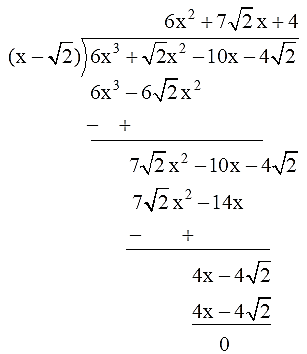6x³+√2x²-10x-4√2= (x-√2) (6x² +7√2x + 4)

By splitting the middle term,

We get,

(x-√2) (6x² + 4√2x + 3√2x + 4)

= (x-√2) [ 2x(3x+2√2) + √2(3x+2√2)]

= (x-√2) (2x+√2)   (3x+2√2)

To get the zeroes of p(x),

Substitute p(x)= 0

(x-√2) (2x+√2)  (3x+2√2)= 0

x= √2 , x= -√2/2 ,x= -2√2/3

Hence, the other two zeroes of p(x) are -√2/2 and -2√2/3

The exemplars for Chapter 2 Polynomials are provided in PDF, which can be downloaded easily. Students can use these solved questions as a reference tool while preparing for the board exam to clear their doubts. These exemplar problems and solutions have been designed by subject experts with respect to the CBSE Syllabus and as per the exercise questions available in the NCERT bookClick here to get exemplars for all chapters.

Students of Class 10 can also go through the online learning materials such as notes, exemplar books, and question papers available in downloadable PDFs to prepare and score well in the board exam. They are also advised to solve sample papers and previous years’ question papers to get an idea of the type of questions asked from the chapter Polynomials and the marking scheme for the same.

## Frequently Asked Questions on NCERT Exemplar Solutions for Class 10 Maths Chapter 2

Q1

### How many questions are present in NCERT Exemplar Solutions for Class 10 Maths Chapter 2?

Chapter 2 of NCERT Exemplar Solutions for Class 10 has four exercises. The first exercise has 5 long answer questions. There is 1 long answer question with many sub-questions in the second exercise. The third exercise has 5 long answer questions, and the fourth exercise has 3 long answer questions. The last exercise covers all the concepts which are discussed in this chapter.
Q2

### Where can I find NCERT Exemplar Solutions for Class 10 Maths Chapter 2 online?

Students can find the NCERT Exemplar Solutions for Class 10 Maths Chapter 2 on BYJU’S website. These NCERT Exemplar Solutions are the top-rated study resources which students can rely on without any hesitation. The PDF of solutions is also available for free download, which can be used by the students without any time constraints. The solutions are curated by the subject experts at BYJU’S strictly based on the latest syllabus of the CBSE Board. Students can speed up their exam preparation using the solutions module, which is available chapter-wise.
Q3

### Are the NCERT Exemplar Solutions for Class 10 Maths Chapter 2 helpful in the board exam preparation?

The NCERT Exemplar Solutions will help students to strengthen their foundation in the basic topics on Maths. The exercise-wise solutions are created by highly knowledgeable experts at BYJU’S, having vast experience in the respective subject. It will help students to focus more and score well in the board exams. The main aim is to boost the confidence level of students and efficiency in solving complex problems quickly.

Download BYJU’S – The Learning App and experience a new method of learning with the help of video lessons explaining the concepts of Maths and other subject topics with the help of visual images and animations.